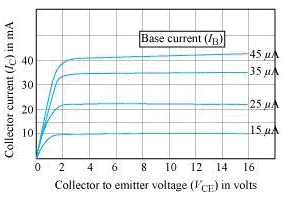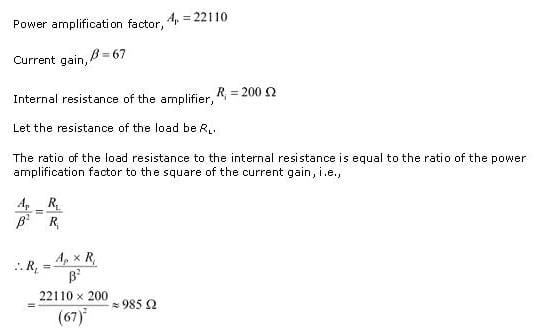NEET  >  Test: Applications of Transistors

# Test: Applications of Transistors

Test Description

## 10 Questions MCQ Test Physics Class 12 | Test: Applications of Transistors

Test: Applications of Transistors for NEET 2023 is part of Physics Class 12 preparation. The Test: Applications of Transistors questions and answers have been prepared according to the NEET exam syllabus.The Test: Applications of Transistors MCQs are made for NEET 2023 Exam. Find important definitions, questions, notes, meanings, examples, exercises, MCQs and online tests for Test: Applications of Transistors below.
Solutions of Test: Applications of Transistors questions in English are available as part of our Physics Class 12 for NEET & Test: Applications of Transistors solutions in Hindi for Physics Class 12 course. Download more important topics, notes, lectures and mock test series for NEET Exam by signing up for free. Attempt Test: Applications of Transistors | 10 questions in 10 minutes | Mock test for NEET preparation | Free important questions MCQ to study Physics Class 12 for NEET Exam | Download free PDF with solutions
 1 Crore+ students have signed up on EduRev. Have you?
Test: Applications of Transistors - Question 1

### Which of the following is not an ac parameter of a transistor?

Detailed Solution for Test: Applications of Transistors - Question 1

Input resistance, Output resistance and Current amplification factor are ac parameters of a transistor.

Test: Applications of Transistors - Question 2

### What is the voltage gain in a common emitter, where input resistance is 3Ω and the load resistance is 24Ω, β = 0.6​

Detailed Solution for Test: Applications of Transistors - Question 2

Voltage gain   =β× (Rc​​/Rb​)
Or,   Voltage gain =0.6×(24/3)​=4.8

Test: Applications of Transistors - Question 3

### An oscillator is nothing but an amplifier with:

Detailed Solution for Test: Applications of Transistors - Question 3

An oscillator is an electronic circuit that converts DC from a power supply to an AC signal. An oscillator produces a periodic and oscillating signal. An oscillator is like an amplifier that gives positive feedback to the circuit. Positive feedback means that a portion of output is combined in phase with the input.

Test: Applications of Transistors - Question 4

The output characteristic for a common emitter transistor is shown in the given figure.Use the graph to find out the current amplification factor for the transistor when VCE = 2 V.​

Test: Applications of Transistors - Question 5

The power amplification for a common emitter (CE) amplifier is 22110. Its current gain is 67 and internal resistance is 200 ohm. What is the value of the of the output resistance?​

Detailed Solution for Test: Applications of Transistors - Question 5Test: Applications of Transistors - Question 6

A common emitter (CE) amplifier has a current gain of 49. The voltage drop across the collector terminal is 1.8 V. The collector resistance is 2.2 kilo-ohm. What is the base current in the CE amplifier?​

Detailed Solution for Test: Applications of Transistors - Question 6

Given,
Current gain(B)=4g
Voltage drop across terminal
Collector=1.8v
Collector resistance=2.2kΩ
Collector current=1.8/2.2x103
Current gain=△IC/△IB
△IB=1.8/2.2x103x4g
(D)△IB=1.67x10-5 A

Test: Applications of Transistors - Question 7

Which of the following relations correctly shows the value of current amplification factor at a constant collector-emitter voltage?

Test: Applications of Transistors - Question 8

Transistors are useful in equipments such as hearing aids and small radio receivers because transistors :

Detailed Solution for Test: Applications of Transistors - Question 8

Transistors consume very less energy and are therefore used in these equipment because these equipment don't have space for big power source.

Test: Applications of Transistors - Question 9

The input voltage applied to a cascaded amplifier (consisting of two amplifiers) is 0.02 V and the output voltage is 6 V. The voltage gain of one of the two amplifiers is 10. What is the voltage gain of the other amplifier?​

Test: Applications of Transistors - Question 10

In a common base transistor circuit, the current gain is 0.98. On changing emitter current by 5 mA, the change in collector current is​

Detailed Solution for Test: Applications of Transistors - Question 10

Given, That the current gain is 0.98
The change in emitter current is Ie​=5mA
Therefore, the change in collector current is Ic​=0.98×Ie​=0.98×5=4.9mA
Therefore, the correct option is C.

## Physics Class 12

157 videos|452 docs|213 tests
 Use Code STAYHOME200 and get INR 200 additional OFF Use Coupon Code
Information about Test: Applications of Transistors Page
In this test you can find the Exam questions for Test: Applications of Transistors solved & explained in the simplest way possible. Besides giving Questions and answers for Test: Applications of Transistors, EduRev gives you an ample number of Online tests for practice

## Physics Class 12

157 videos|452 docs|213 tests

### How to Prepare for NEET

Read our guide to prepare for NEET which is created by Toppers & the best Teachers